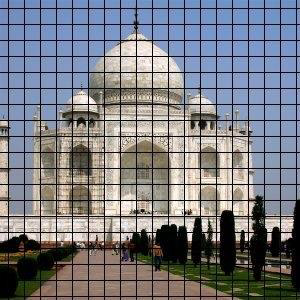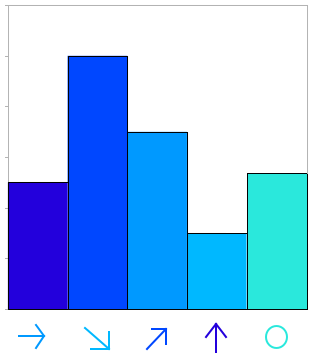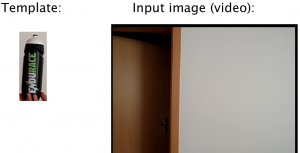Posted on

# Dominant Orientation Templates## Description

Dominant orientation templates (DOT) is a method for real-time object detection, which works well for detection of untextured objects and is related to method Histogram of oriented gradients. DOT is neither based on statistical learning of objectâ€™s shapes nor on feature point detection, but it uses real-time Template Matching recognition with locally most dominant orientations from HoG.

## OpenCV function used

cvCaptureFromAVI, cvtColor

## The process

1. Computation of gradients for each pixel in template and input image
1. Provided by convolution kernel
2. For each pixel
3. Gradient is defined by magnitude and direction
4. 0-180Â° instead of 0-360Â° range
5. Directions can be discretized from 0-180Â° into bins (e.g. 9 bins by 20Â° )
```for (int r=0;r<=area.rows-region_size;r+=region_size)
{
for (int s=0;s<=area.cols-region_size;s+=region_size)
{
if (mag>min_magnitude)
template_hist.hist_matrix[hx][hy].bins[n0]+=mag;
}
}
```
2. Dividing pixels into regions```for (int r=0;r<=area.rows-region_size;r+=region_size)
// moving in the picture with step size of 7 or 9
```
3. Computing most dominant gradient orientations for each region```if (template_hist.hist_matrix[i][j].bins[k]>max) //now only the most dominant
{
Â  Â  ifÂ  (template_hist.hist_matrix[i][j].bins[k]>min_magnitude)
Â  Â  {
Â  Â  Â  Â  max=template_hist.hist_matrix[i][j].bins[k];
Â  Â  Â  Â  max_index=k;
Â  Â  }
}
```
4. Template matching and comparing of most dominant orientations.
5. Evaluating comparison.